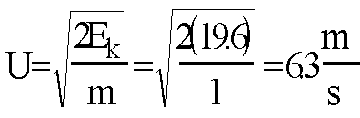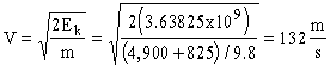+ Text Only Site
+ Non-Flash Version
+ Contact GlennEnergy Activity Answers A model glider has a mass of 1 kg. How much potential energy does it have 2 meters off the ground? Ep = mgh = (1)(9.8)(2) = 19.6 j The same model has a velocity of 2.2 m/s. How much kinetic energy does it have? Ek = 1/2(m)V2 = (1/2)(1)(2.2)2 = 2.42 j If the same model descends 2 meters and all it's potential energy is converted to kinetic energy, what is the glider's change in velocity? Ek = 1/2(m)U2A full-sized glider has a weight of 4,900 N, while it's pilot has a weight of 825 N. If it is 1,000 meters off the ground, how much potential energy do the plane and pilot have? Ep = mgh or Fwh = (4,900 + 825)(1,000) = 5,725,000 j The same glider from Problem 4 has a velocity of 35 m/s. How much kinetic energy does it have? Ek = 1/2(m)V2 = 1/2 [(4.900 + 825)/9.8](352) = 357,813 j The same glider from Problem 4 has a velocity of 35 m/s. The glider descends 900 meters. What is it's new velocity? Ep = Fwh = (4,900 + 825)(900) = 3.63825 * 109 jNew Vel. = Old + Change = 35 + 132 = 167 m/s Compare the velocity you calculated in Problem 6 to the speed of sound. Is this answer reasonable? Why or why not? 167/346 = .48 Approximately 1/2 the speed of sound. (Note: This speed is faster than a B-17 Flying Fortress.)

Related Pages:
Standards
Activity
Worksheet
Lesson Index
Aerodynamics Index+ Inspector General Hotline + Equal Employment Opportunity Data Posted Pursuant to the No Fear Act + Budgets, Strategic Plans and Accountability Reports + Freedom of Information Act + The President's Management Agenda + NASA Privacy Statement, Disclaimer, and Accessibility CertificationEditor: Tom Benson NASA Official: Tom Benson Last Updated: Thu, May 13 02:38:26 PM EDT 2021 + Contact Glenn